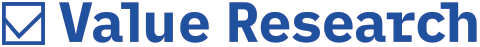# Role of Standard Deviation

##### Shweta wants to know why the standard deviation mentioned in the fact sheets varies from that in our scorecard

Why the standard deviation, alpha etc. mentioned in the fact sheet of mutual funds vary from the data available in your scorecard? Standard deviation measures the deviation from mean. But what does a standard deviation of 28 implies? Is it in per cent? Is the calculation made, on an annualized basis?
- Shweta

Standard Deviation, Alpha, Beta etc are statistical measurements which help investors determine the risk-reward profile of a mutual fund.
There can be a difference in the values of standard deviation, alpha, etc in our scorecard, as compared to those in the fact sheets. This is because of two reasons - the time span considered for calculation of the average return and the frequency of calculation. For instance, Value Research calculates standard deviation (S.D.) for equity funds on the basis of trailing three years, monthly returns, whereas for debt and gilt funds it is based on the weekly returns over the past 18 months. The time span or frequency for calculation of standard deviation would be different between fund fact sheets and the scorecard, hence resulting in the discrepancy.
Standard Deviation of a fund depicts, that how much the returns of the fund have deviated from the mean level. The higher the value of standard deviation, the greater will be the volatility in the fund's returns. Standard deviation of 28 means that the fund's return can fluctuate in either direction (up or down) by 28 per cent from its average return. These values are annualized and updated on monthly basis.